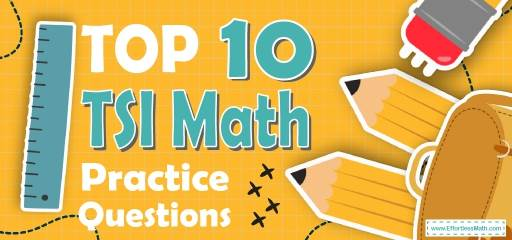# Top 10 TSI Math Practice QuestionsTaking the TSI Math test in a few weeks or even in a few days? The best way to prepare for your TSI Math test is to work through as many TSI Math practice questions as possible. Here are the top 10 TSI Math practice questions to help you review the most important TSI Math concepts. These TSI Math practice questions are designed to cover mathematics concepts and topics that are found on the actual test. The questions have been fully updated to reflect the latest 2022 TSI guidelines. Answers and full explanations are provided at the end of the post.

Start your TSI Math test prep journey right now with these sample TSI Math questions.

## TSI Math Practice Questions

1- $$(x + 7) (x + 5) = ?$$

A. $$x^2 + 12x + 12$$

B. $$2x + 12x + 12$$

C. $$x^2 + 35x + 12$$

D. $$x^2 + 12x + 35$$

2- If $$x$$ is a positive integer divisible by 6, and $$x<6$$, what is the greatest possible value of $$x$$?

A. 54

B. 48

C. 36

D. 59

3- $$x^2 – 81 = 0$$ , $$x$$ could equal to:

A. 6

B. 9

C. 12

D. 15

4- If $$a = 8$$, what is the value of $$b$$ in this equation?
$$b=\frac{a^2}{4}+4$$

A. 24

B. 22

C. 20

D. 28

5- If $$6.5 < x ≤ 9.0$$, then $$x$$ cannot be equal to:

A. 6.5

B. 9

C. 7.2

D. 7.5

6- What is the area of an isosceles right triangle that has one leg that measures 6 cm?

A. 18 cm

B. 36 cm

C. 6 $$\sqrt{2}$$ cm

D. 72 cm

7- Which of the following expressions is equivalent to $$10 – (\frac{2}{3}) x ≥ 12$$

A. $$x ≥ –3$$

B. $$x ≤ –3$$

C. $$x ≥ 24 \frac{1}{3}$$

D. $$x ≤24 \frac{1}{3}$$

8- Which of the following is a factor of both $$x^2 – 2x – 8$$ and $$x^2 – 6x + 8$$?

A. $$(x – 4)$$

B. $$(x +4)$$

C. $$(x – 2)$$

D. $$(x +2)$$

9- $$\frac{1}{6b^2} + \frac{1}{6b} = \frac{1}{b^2}$$, then $$b = ?$$

A. $$-\frac{16}{15}$$

B. 5

C. $$-\frac{15}{16}$$

D. 8

10- If two angles in a triangle measure 53 degrees and 45 degrees, what is the value of the third angle?

A. 8 degrees

B. 42 degrees

C. 82 degrees

D. 98 degrees

## Best TSI Math Prep Resource for 2022

1- D
Use FOIL (First, Out, In, Last)
$$(x + 7) (x + 5) = x^2 + 5x + 7x + 35 = x^2 + 12x + 35$$

2- A
$$\frac{54}{6}=\frac{27}{3}=9$$
$$\frac{48}{6}=\frac{24}{3}= 8$$
$$\frac{36}{6}=\frac{18}{3}=6$$
$$\frac{59}{6}=\frac{59}{6}$$
59 is prime number

3- B
$$x^2 – 81 = 0$$ ⇒ $$x^2 = 81$$ ⇒ $$x = 9$$

4- C
If $$a = 8$$ then $$b =\frac{8^2}{4} + 4$$ ⇒ $$b = \frac{64}{4} + 4$$ ⇒ $$b = 16 + 4 = 20$$

5- A
If $$6.5 < x ≤ 9.0$$, then x cannot be equal to 6.5

6- A
$$a=6$$⇒ area of triangle is $$=\frac{1}{2} (6×6)=\frac{36}{2}=18$$ cm

7- B
Simplify:
$$10 – \frac{2}{3} x ≥ 12$$ ⇒$$– \frac{2}{3}2 x ≥ 2$$ ⇒ $$– x ≥ 3 ⇒ x ≤ – 3$$

8- A
Factor each trinomial $$x^2 – 2x – 8$$ and $$x^2 – 6x + 8$$
$$x^2 – 2x – 8$$ ⇒ $$(x – 4)(x + 2)$$
$$x^2 – 6x + 8$$ ⇒ $$(x – 2)(x – 4)$$

9- B

$$\frac{1+b}{6b^2}=\frac{1}{b^2}$$
⇒$$(b≠0) b^2+b^3=6b^2$$⇒$$b^3-5b^2=0$$⇒$$b^2 (b-5)=0$$⇒$$b-5=0⇒b=5$$

10- C
$$53^\circ + 45^\circ = 98^\circ$$
$$180^\circ – 98^\circ = 82^\circ$$
The value of the third angle is $$82^\circ$$.

Looking for the best resource to help you succeed on the TSI Math test?

## More from Effortless Math for TSI Test …

### Does the anxiety of taking the TSI math test bother you?

Anxiety is useless because we at Top 10 Tips to Overcome TSI Math Anxiety will teach you tips that will ease your mind!

### Can’t focus on studying well without having a study plan for the TSI math test?

Before preparing a study plan, hold on for a few minutes and spend time reading the Top 10 Tips to Create a TSI Math Study Plan.

### Need TSI math worksheets that organize useful tips perfectly into questions?

TSI Math Worksheets: FREE & Printable at your disposal for this purpose.

## Have any questions about the TSI Test?

### What people say about "Top 10 TSI Math Practice Questions - Effortless Math: We Help Students Learn to LOVE Mathematics"?

No one replied yet.

X
52% OFF

Limited time only!

Save Over 52%

SAVE $40 It was$76.99 now it is \$36.99